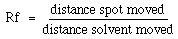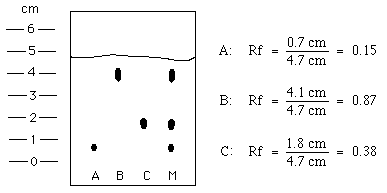Retention Factors

## Retention Factors

The amount that each component of a mixture travels can be quantified using retention factors (Rf). The retention factor of a particular material is the ratio of the distance the spot moved above the origin to the distance the solvent front moved above the origin. It can be calculated using the formula:The Rf values for each of the components in the previous example can be calculated:Notice that (1) the bigger the Rf, the further the spot moved and (2) that the Rf should be the same for a component regardless of how far the solvent moves.

Retention factors are useful in comparing the results of one chromatogram to the results of another. If the conditions in which the chromatogram are run are unchanged (same mobile and stationary phases), the retention factor for a given material should remain constant. This allows unknowns to be compared to known materials. If the retention factor of an unknown does not match that of a known material, they are not the same compound. Similar retention factors suggest that the two samples could be the same, but is not proof. In reality, the retention factors will vary slightly from sample to sample. Interactions of the individual components with each other and the concentration of the component in the sample will both affect the Rf value.